Updating search results...

# 90 Results

View
Selected filters:
• Mudd Math Fun Facts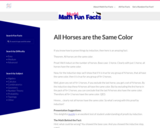Restricted Use
Rating
0.0 stars

This Math Fun Fact focuses on proof by induction.
Math Fun Facts were developed as warm-up activities. They are mathematical tidbits meant to arouse curiosity and fascination with the subject. Fun Facts give students a glimpse that mathematics is full of interesting ideas, patterns, and new modes of thinking.

Subject:
Mathematics
Material Type:
Activity/Lab
Provider:
Mudd Math Fun Facts
Author:
Francis Su
12/01/2022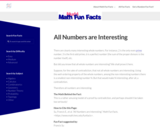Restricted Use
Rating
0.0 stars

This Math Fun Fact is a rather amusing model of a proof by contradiction, and perhaps shouldnÕt be taken too seriously!
Math Fun Facts were developed as warm-up activities. They are mathematical tidbits meant to arouse curiosity and fascination with the subject. Fun Facts give students a glimpse that mathematics is full of interesting ideas, patterns, and new modes of thinking.

Subject:
Mathematics
Material Type:
Activity/Lab
Provider:
Mudd Math Fun Facts
Author:
Francis Su
12/01/2022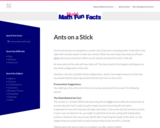Restricted Use
Rating
0.0 stars

This Math Fun Fact focuses on seeking alternate ways to look at a problem.
Math Fun Facts were developed as warm-up activities. They are mathematical tidbits meant to arouse curiosity and fascination with the subject. Fun Facts give students a glimpse that mathematics is full of interesting ideas, patterns, and new modes of thinking.

Subject:
Mathematics
Material Type:
Activity/Lab
Provider:
Mudd Math Fun Facts
Author:
Francis Su
12/01/2022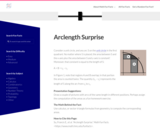Restricted Use
Rating
0.0 stars

This Math Fun Fact focuses on using calculus, or sector-triangle formulas from geometry, to compute corresponding areas.
Math Fun Facts were developed as warm-up activities. They are mathematical tidbits meant to arouse curiosity and fascination with the subject. Fun Facts give students a glimpse that mathematics is full of interesting ideas, patterns, and new modes of thinking.

Subject:
Mathematics
Material Type:
Activity/Lab
Provider:
Mudd Math Fun Facts
Author:
Francis Su
12/01/2022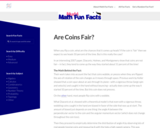Restricted Use
Rating
0.0 stars

This Math Fun Fact focuses on the theoretical model of coin flipping.
Math Fun Facts were developed as warm-up activities. They are mathematical tidbits meant to arouse curiosity and fascination with the subject. Fun Facts give students a glimpse that mathematics is full of interesting ideas, patterns, and new modes of thinking.

Subject:
Mathematics
Material Type:
Activity/Lab
Provider:
Mudd Math Fun Facts
Author:
Francis Su
12/01/2022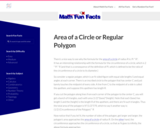Restricted Use
Rating
0.0 stars

This Math Fun Fact focuses on approximating the area of the circle by slicing it into thin wedges, which is analogous to the process of integration (in calculus) to find an area.
Math Fun Facts were developed as warm-up activities. They are mathematical tidbits meant to arouse curiosity and fascination with the subject. Fun Facts give students a glimpse that mathematics is full of interesting ideas, patterns, and new modes of thinking.

Subject:
Mathematics
Material Type:
Activity/Lab
Provider:
Mudd Math Fun Facts
Author:
Francis Su
12/01/2022Restricted Use
Rating
0.0 stars

This Math Fun Fact focuses on integration in polar coordinates.
Math Fun Facts were developed as warm-up activities. They are mathematical tidbits meant to arouse curiosity and fascination with the subject. Fun Facts give students a glimpse that mathematics is full of interesting ideas, patterns, and new modes of thinking.

Subject:
Mathematics
Material Type:
Activity/Lab
Provider:
Mudd Math Fun Facts
Author:
Francis Su
12/01/2022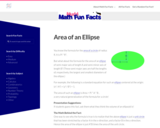Restricted Use
Rating
0.0 stars

This Math Fun Fact focuses on the area of an ellipse.
Math Fun Facts were developed as warm-up activities. They are mathematical tidbits meant to arouse curiosity and fascination with the subject. Fun Facts give students a glimpse that mathematics is full of interesting ideas, patterns, and new modes of thinking.

Subject:
Mathematics
Material Type:
Activity/Lab
Provider:
Mudd Math Fun Facts
Author:
Francis Su
12/01/2022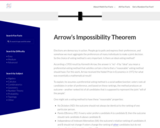Restricted Use
Rating
0.0 stars

This Math Fun Fact focuses on ArrowÕs Impossibility Theorem.
Math Fun Facts were developed as warm-up activities. They are mathematical tidbits meant to arouse curiosity and fascination with the subject. Fun Facts give students a glimpse that mathematics is full of interesting ideas, patterns, and new modes of thinking.

Subject:
Mathematics
Material Type:
Activity/Lab
Provider:
Mudd Math Fun Facts
Author:
Francis Su
12/01/2022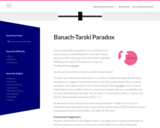Restricted Use
Rating
0.0 stars

This Math Fun Fact focuses on the Banach-Tarski paradox.
Math Fun Facts were developed as warm-up activities. They are mathematical tidbits meant to arouse curiosity and fascination with the subject. Fun Facts give students a glimpse that mathematics is full of interesting ideas, patterns, and new modes of thinking.

Subject:
Mathematics
Material Type:
Activity/Lab
Provider:
Mudd Math Fun Facts
Author:
Francis Su
12/01/2022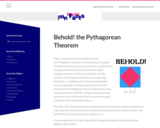Restricted Use
Rating
0.0 stars

This Math Fun Fact focuses on the Pythagorean theorem.
Math Fun Facts were developed as warm-up activities. They are mathematical tidbits meant to arouse curiosity and fascination with the subject. Fun Facts give students a glimpse that mathematics is full of interesting ideas, patterns, and new modes of thinking.

Subject:
Mathematics
Material Type:
Activity/Lab
Provider:
Mudd Math Fun Facts
Author:
Francis Su
12/01/2022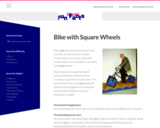Restricted Use
Rating
0.0 stars

This Math Fun Fact focuses on the fundamental differential equation.
Math Fun Facts were developed as warm-up activities. They are mathematical tidbits meant to arouse curiosity and fascination with the subject. Fun Facts give students a glimpse that mathematics is full of interesting ideas, patterns, and new modes of thinking.

Subject:
Mathematics
Material Type:
Activity/Lab
Provider:
Mudd Math Fun Facts
Author:
Francis Su
12/01/2022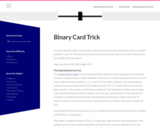Restricted Use
Rating
0.0 stars

This Math Fun Fact focuses on the properties of binary numbers.
Math Fun Facts were developed as warm-up activities. They are mathematical tidbits meant to arouse curiosity and fascination with the subject. Fun Facts give students a glimpse that mathematics is full of interesting ideas, patterns, and new modes of thinking.

Subject:
Mathematics
Material Type:
Activity/Lab
Provider:
Mudd Math Fun Facts
Author:
Francis Su
12/01/2022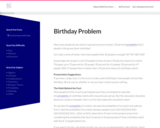Restricted Use
Rating
0.0 stars

This Math Fun Fact focuses on calculating the probability of a match.
Math Fun Facts were developed as warm-up activities. They are mathematical tidbits meant to arouse curiosity and fascination with the subject. Fun Facts give students a glimpse that mathematics is full of interesting ideas, patterns, and new modes of thinking.

Subject:
Mathematics
Material Type:
Activity/Lab
Provider:
Mudd Math Fun Facts
Author:
Francis Su
12/01/2022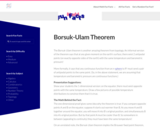Restricted Use
Rating
0.0 stars

This Math Fun Fact focuses on the Borsuk-Ulam theorem.
Math Fun Facts were developed as warm-up activities. They are mathematical tidbits meant to arouse curiosity and fascination with the subject. Fun Facts give students a glimpse that mathematics is full of interesting ideas, patterns, and new modes of thinking.

Subject:
Mathematics
Material Type:
Activity/Lab
Provider:
Mudd Math Fun Facts
Author:
Francis Su
12/01/2022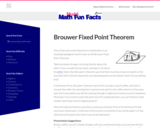Restricted Use
Rating
0.0 stars

This Math Fun Fact focuses on the Brouwer Fixed Point Theorem.
Math Fun Facts were developed as warm-up activities. They are mathematical tidbits meant to arouse curiosity and fascination with the subject. Fun Facts give students a glimpse that mathematics is full of interesting ideas, patterns, and new modes of thinking.

Subject:
Mathematics
Material Type:
Activity/Lab
Provider:
Mudd Math Fun Facts
Author:
Francis Su
12/01/2022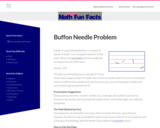Restricted Use
Rating
0.0 stars

This Math Fun Fact focuses on calculating pi and probability theory.
Math Fun Facts were developed as warm-up activities. They are mathematical tidbits meant to arouse curiosity and fascination with the subject. Fun Facts give students a glimpse that mathematics is full of interesting ideas, patterns, and new modes of thinking.

Subject:
Mathematics
Material Type:
Activity/Lab
Provider:
Mudd Math Fun Facts
Author:
Francis Su
12/01/2022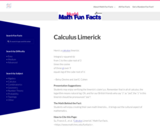Restricted Use
Rating
0.0 stars

This Math Fun Fact focuses on a calculus limerick.
Math Fun Facts were developed as warm-up activities. They are mathematical tidbits meant to arouse curiosity and fascination with the subject. Fun Facts give students a glimpse that mathematics is full of interesting ideas, patterns, and new modes of thinking.

Subject:
Mathematics
Material Type:
Activity/Lab
Provider:
Mudd Math Fun Facts
Author:
Francis Su
12/01/2022Restricted Use
Rating
0.0 stars

This Math Fun Fact focuses on the theory of countable and uncountable sets.
Math Fun Facts were developed as warm-up activities. They are mathematical tidbits meant to arouse curiosity and fascination with the subject. Fun Facts give students a glimpse that mathematics is full of interesting ideas, patterns, and new modes of thinking.

Subject:
Mathematics
Material Type:
Activity/Lab
Provider:
Mudd Math Fun Facts
Author:
Francis Su
12/01/2022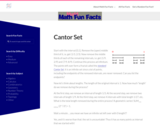Restricted Use
Rating
0.0 stars

This Math Fun Fact focuses on real numbers and the standard Cantor Set.
Math Fun Facts were developed as warm-up activities. They are mathematical tidbits meant to arouse curiosity and fascination with the subject. Fun Facts give students a glimpse that mathematics is full of interesting ideas, patterns, and new modes of thinking.

Subject:
Mathematics
Material Type:
Activity/Lab
Provider:
Mudd Math Fun Facts
Author:
Francis Su